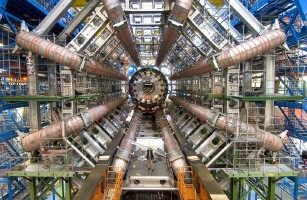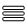Magnets & Detectors I

Taking a closer look at LHC

 The Lorentz Force plays another very important role in LHC. It is responsible for curving the trajectory of the new particles created after the proton collisions. According to electrical charge, mass and energy,  particles trajectory will be curved to be analysed separately. The imaxe shows the generation of a Higgs particle followed of two photons which are not affected for the magnetic field. Each detector has its own design for that magnetic field, and it´s worth taking a look at CMS and ATLAS.The CMS (Compact Muon Solenoid) detector is a 12500 tonne instrument (the magnetic return yoke contains more iron than the Eiffel Tower). Its magnet consists mainly of three parts: a superconducting coil, a vacuum tank and the magnet yoke. The solenoid produces an axial field whereas the yoke is responsible for the return of the magnetic flux. Due to the general design of the CMS detector, the yoke is split into a cylindrical central part, the barrel, and at the extremities, two endcaps made of 600 mm thick disks.The Solenoid consists of 5 distinct modules(2,5 m length) Each module consists of an aluminium cylinderwith four internal layers of winding, each with 109 turns.  So that:  N = 5·4·109 = 2180 turns. The total length of solenoid is:  L = 5·2,5 m =12,5 m The current intensity will be  I = 19500 A. So:     B = μ0·N·I/L    ⇒   B ≈ 4 TWe can also calculate the CMS Solenoide Inductance.

The solenoid has 2180 turns , the magnetic field is 3,8 T and the surface is S = π·32=28,3 m2.

So, themagnetic Flux throughout the surface is:

φ = N·B·S     φ = 2180·3,7·28,3      φ ≈ 230 kWb

With,    φ = L·I   ⇒   L = 230000/19500      L  12 H

The total stored energy is:

E = ½·L·I2   ⇒   E ≈ 2,3 GJ

equivalent to about half-a-tonne of TNT.

The ATLAS detector features a hybrid system of four superconducting magnets: a Central Solenoid surrounded by 2 End-cap Toroids and a Barrel Toroid. The magnet system dimensions are 20 m in diameter and 26 m in length. With its 2 GJ stored energy in air, it actually is the largest superconducting magnet in the world.

 The central solenoid, which is 5.5 tonnes in weight, 2.5 m in diameter and 5.3 m in length, provide an axial magnetic field of 2 T at the centre of the ATLAS tracking volume. As the solenoid coil precedes the barrel liquid-argon (LAr) electromagnetic calorimeter, its thickness must be minimized to achieve maximal calorimeter performance. It contains 9 km of superconducting wire cooled by liquid helium and an electric current of 8000 Amperes.With 7 km of superconducing wire:

Nº of turns=7000/(π·2,5)=1142 turns.

Since  B = μ0·N·I/L  ⇒   B = (4π·10-7·1142·8000)/5,3   ⇒   B ≈ 2 T

φ = N·B·S ⇒ φ = 1142·2·( π·1,252)    φ ≈ 11200 Wb

With, φ = L·I     L = 11200/8000 ⇒ L ≈ 1,4 H

The stored energy is:    E = ½·L·I2 ⇒ E ≈ 44,8 MJ

 The ATLAS detector also requires a large superconducting Barrel Toroid (BT) with overall dimensions of 25 m length and 22 m diameter. The Barrel Toroid provides the magnetic field for the muon detector. The toroid is assembled from 8 flat race track coils of dimensions 25m x 5m. They were successively inserted in the underground cavern and assembled as a full toroid by using 16 supporting rings of struts that link the 8 coils to form a rigid and stable structure. The total mass of the toroid is 850 t.The barrel toroid system contains over 100 km of superconducting wire, and a current design of 20500 A.

Every flat race track coil is over (25+25+5+5) ~ 60 m length. Since ther are 100 km superconducting wire in the toroid we can consider :

Nº equivalent Turns = 100000/60      Nº Turns ≈ 1670
Since  B = μ0·N·I/L     B = (4π·10-7·1670·20500)/(2π·8,5)

B ≈ 1 T

φ = N·B·S ⇒ φ = 1670·1·( 25·5) ⇒ φ ≈ 208750 Wb

With, φ = L·I      L = 208750/20500 ⇒ L ≈ 10,2 H

The stored energy is:    E = ½·L·I2 ⇒ E ≈ 2 GJ

The Airbus 380 take-off speed, under typical conditions, is around 280 km/h (~78 m/s), and its maximum take-off weight is about 600 tonnes.

So,
EK = ½·m·v2   ⇒  EK = ½·600000·782
EK ~ 2 GJ